Here we take a banana graph with 2 vertices and 3 edges. The integer `d' is the flow into the graph (and thus also the flow out at the other vertex). The base is (locally) affine space of dimension 3. We think of it as a toric variety, with fan the positive orthant in R^3 (a single cone).

The picture shows the fan of Mhat, a slice taken at x+y+z = 1 (because it is easier to draw in 3d). Thus the fan consists of the boundary of the positive orthant, together with a bunch of rays (1-dimensional cones), which appear as dots because we have taken this slice.

It would be nice to find a canonical way to compactify this. Compactification corresponds to cutting up the big triangle into convex polygons (ideally smaller triangles...) such that every green dot appears at a vertex. It is clear that it is possible to do this in an `ad hoc' way, but can it be made canonical?!

Sage code to produce these pictures is here

d=1: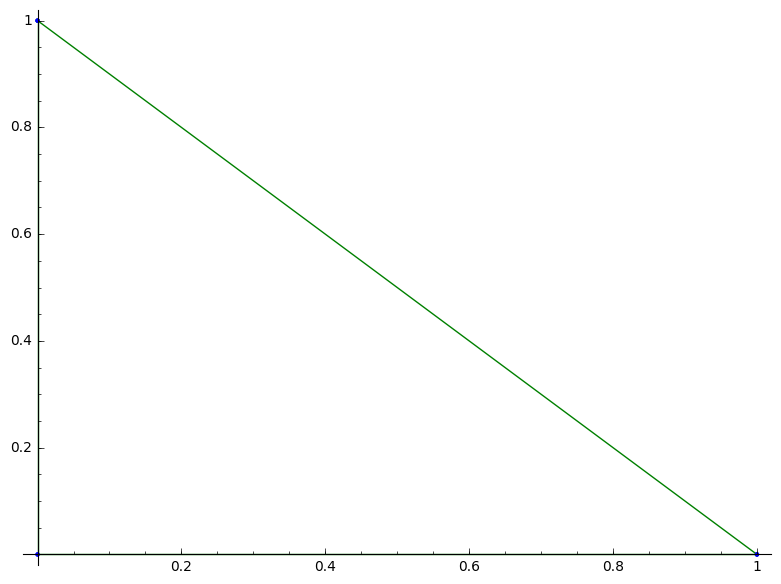d=2: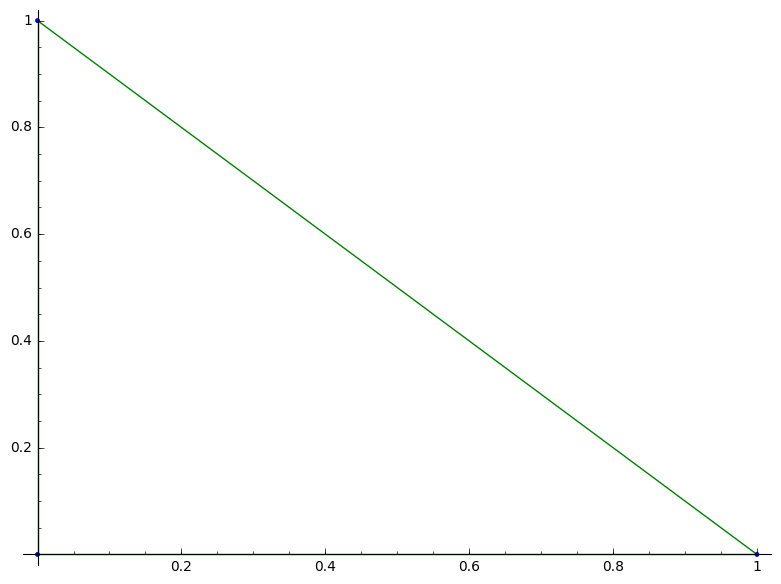d=2#: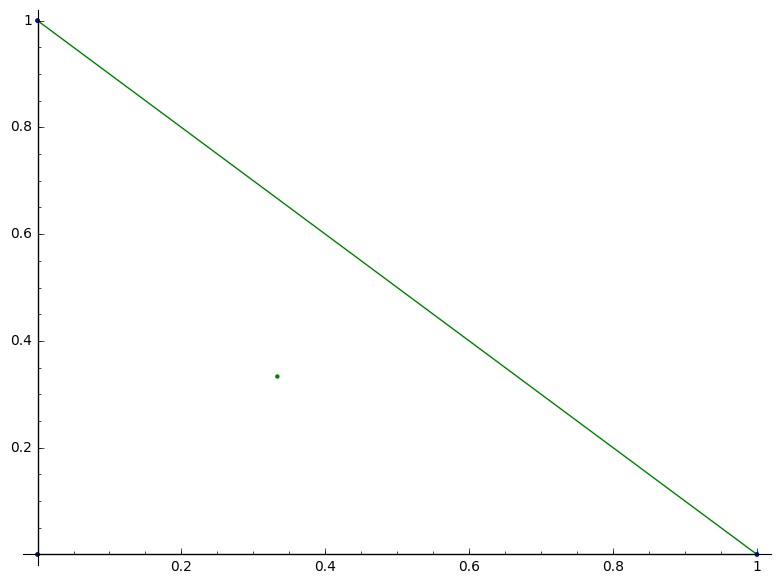d=4: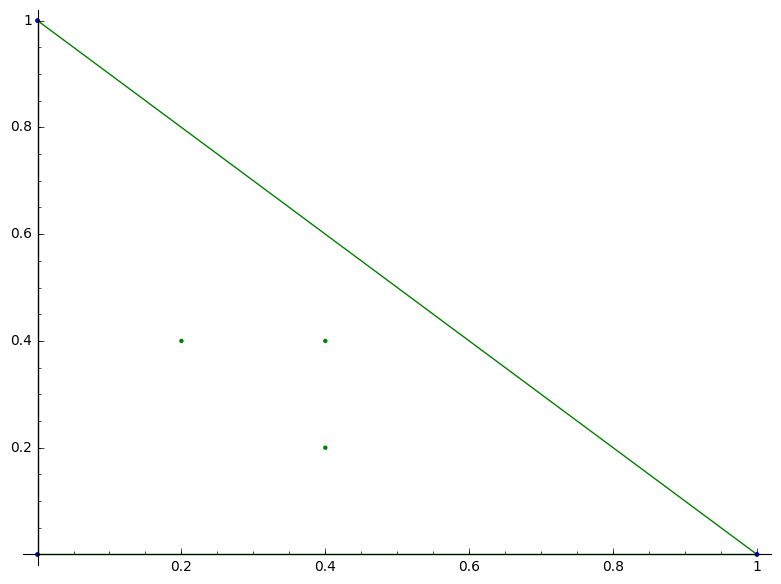d=5:d=6: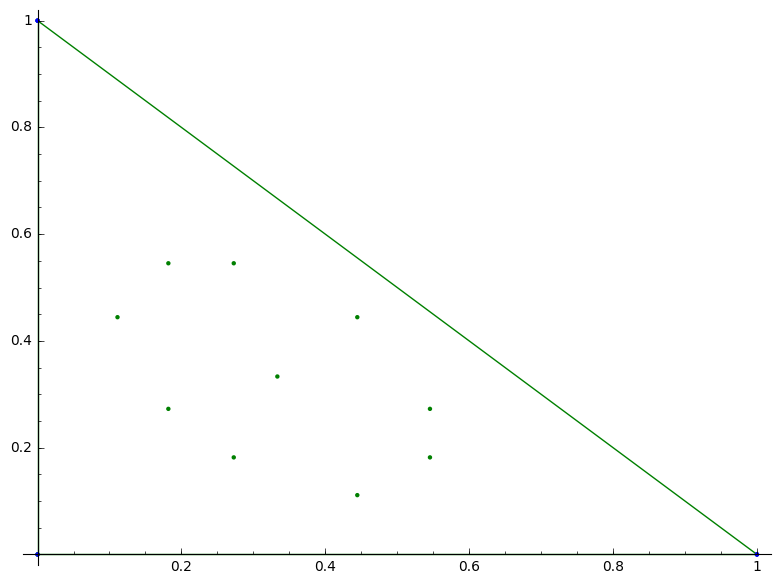d=7: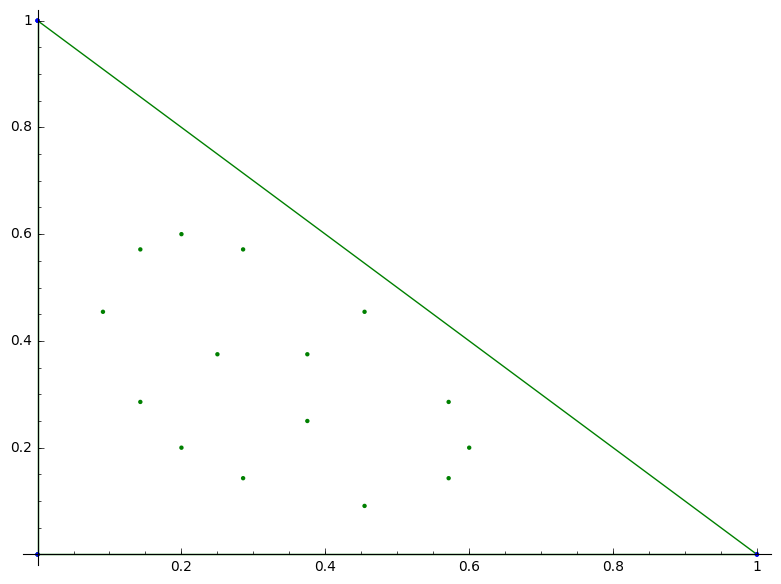d=8: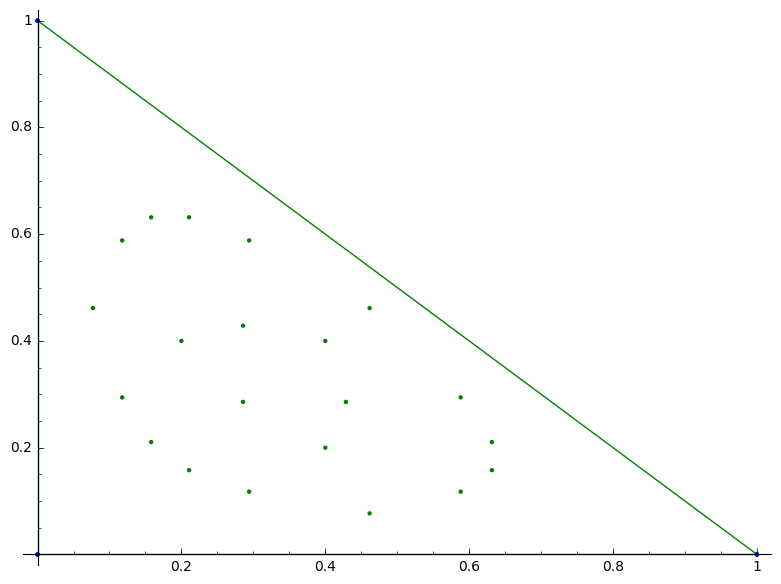d=9:d=10: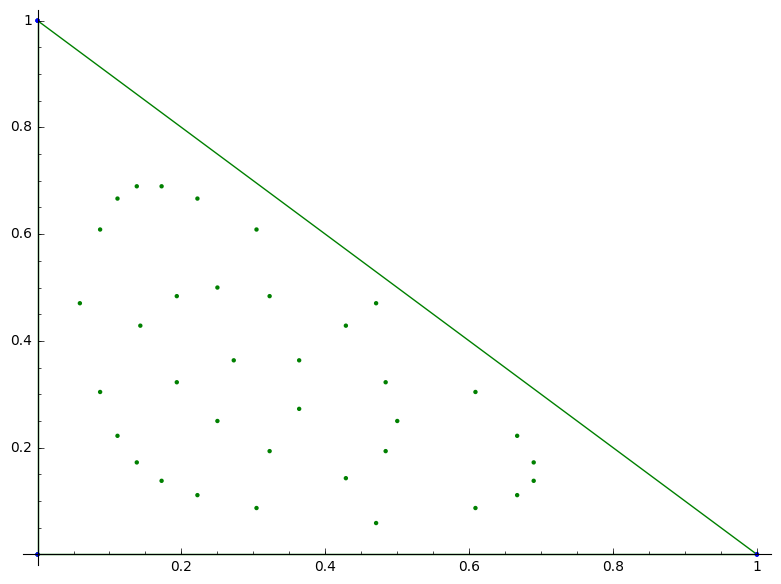d=11:d=12: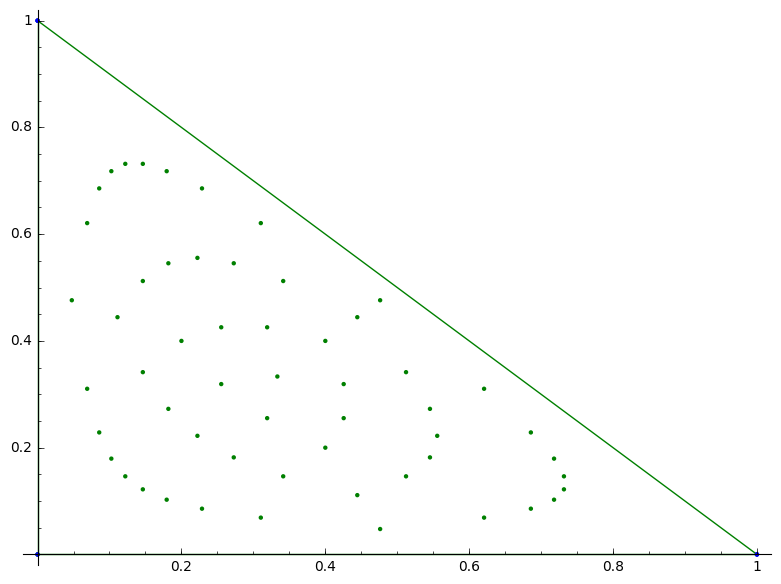d=13: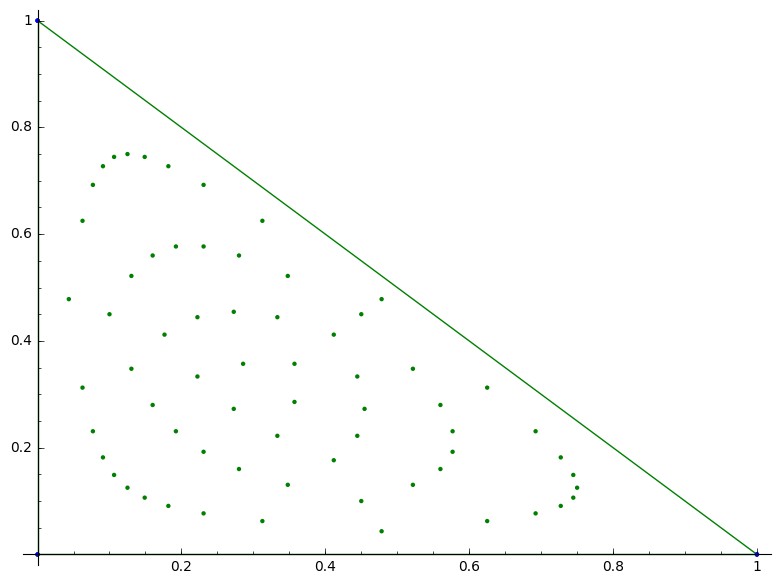d=14: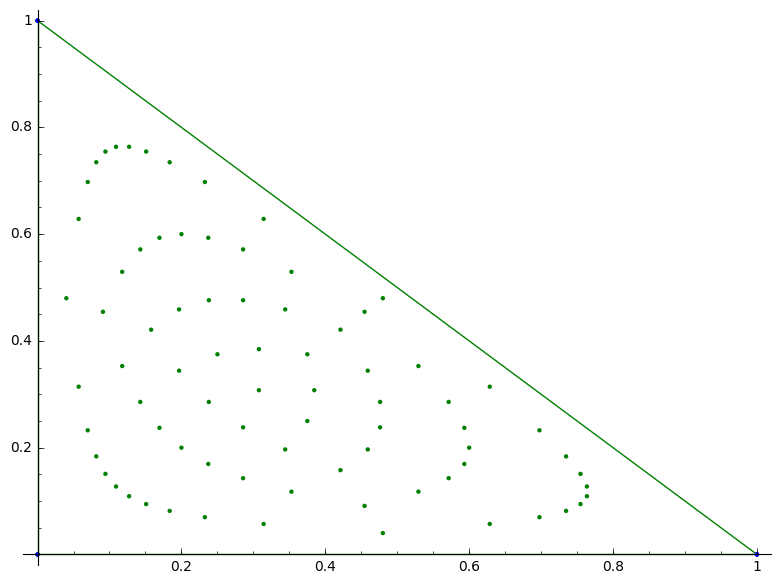d=15:d=20:d=25:d=30: